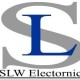# Wave reflection – why is it important to understand this concept in RF design?

### Wave reflection – why is it important to understand this concept in RF design?

[Introduction]For non-RF engineers, this article briefly introduces a term related to a key characteristic inherent in RF design: wave reflection. The key difference between ordinary circuits operating at low frequencies and circuits designed for RF frequencies is their electrical size. RF designs can be sized at multiple wavelengths, resulting in Voltage and current magnitudes and phases that vary with the physical dimensions of the components.This provides some basic core principle properties for the design and analysis of RF circuits1.

problem:

Wave reflection – why is it important to understand this concept in RF design?

This article, aimed at non-RF engineers, briefly introduces a term related to a key characteristic inherent in RF design: wave reflection. The key difference between ordinary circuits operating at low frequencies and circuits designed for RF frequencies is their electrical size. RF designs can be sized at multiple wavelengths, resulting in voltage and current magnitudes and phases that vary with the physical dimensions of the components. This provides some basic core-principle properties for the design and analysis of RF circuits1.

Basic Concepts and Terminology

Suppose a transmission line (such as coaxial cable or microstrip) is terminated with an arbitrary load, and the wave quantities a and b are defined, as shown in Figure 1.Figure 1. A transmission line terminated with a matched signal source with a single-port load.

These wave quantities are the complex amplitudes of the voltage waves incident on and reflected from the load. We can now use these quantities to define the voltage reflection coefficient Γ, which describes the ratio of the complex amplitude of the reflected wave to the complex amplitude of the incident wave:The reflection coefficient can also be used for the transmission line Z0The characteristic impedance and load ZLThe complex input impedance of is expressed as:RF engineering generally adopts Z0 = 50 Ω, which is a compromise between signal attenuation and power handling capacity, which can be achieved with coaxial transmission lines. However, in some applications, such as in broadcast systems where RF signals need to be transmitted over long distances, Z0 = 75 Ω is a more common choice to reduce cable losses.

Regardless of the value of the characteristic impedance, if the load impedance is the same (ZL = Z0), it means that the load matches the transmission line. It should be noted that this condition is valid only when the signal source matches the transmission line, as shown in Figure 1, and this assumption is also made in this paper. In this case, no reflected waves are generated (Γ = 0), the load receives the maximum power from the source, and in the case of total reflection (|Γ| = 1), no wave is delivered to the load at all power.

If the load does not match (ZL ≠ Z0), the full incident power will not be received. The corresponding power “loss” is called return loss (RL), which is related to the magnitude of the reflection coefficient and can be expressed as:Return loss is the ratio of the incident power at the load to the power reflected back. Return loss is always a non-negative value and represents how well the load matches the network impedance “shown” on the load towards the source.

If the loads are not matched, the presence of reflected waves can cause standing waves, which can cause unstable voltage amplitudes that vary with line position. The parameter used to quantify line impedance mismatch is called the standing wave ratio (SWR) and is defined as:Since we usually resolve SWR in terms of maximum and minimum voltages, this quantity is also known as Voltage Standing Wave Ratio (VSWR). SWR is a real number ranging from 1 to infinity, where SWR = 1 means load matching.

in conclusion

RF circuits have many fundamental characteristics that differ from ordinary circuits. Designing and analyzing microwave circuits requires the use of extended concepts to solve practically relevant problems. This article introduces and discusses some important concepts and terminology related to wave reflection, a major characteristic of RF systems.

Analog Devices offers a broad portfolio of RF integrated circuits with deep system design expertise to meet the demanding requirements of a wide range of RF applications. In addition, ADI provides an entire ecosystem of technical support, including design tools, simulation models, reference designs, rapid prototyping platforms and technical forums, to help RF engineers simplify the development process of target applications.

References

1 MS Gupta. “What is RF?” IEEE Microwave Journal, Volume 2, Issue 4, December 2001.

Hiebel, Michael. Fundamentals of Vector Network Analysis. Rohde & Schwarz, 2007.

Pozar, David M. Microwave Engineering, 4th ed. Wiley, 2011.

The Links:   SKM75GD123D G190ETN01-204